# Nomography

Also found in: Dictionary, Thesaurus, Medical, Wikipedia.

## Nomography

a branch of mathematics that combines the theory and practical methods of constructing nomographs, or nomograms—special charts representing functional relations. Each chart represents a defined range of change of variables, and each of the values of the variables in this range is represented on the nomograph by a specific geometric element (a point or line). The values of variables connected by a functional relation are represented in a specific correspondence that is common to nomographs of the same type.

Figure 1 illustrates a sample nomograph for calculating αy—one of the cutting angles on a tool-cutting machine for the given values of the cutting angles α and ϕ. The relation between these quantities is defined by the formula

tan αy = tan α/sin ϕ

The nomograph consists of three scales: the scales of angles αy, α, and ϕ. The points on each of the scales represent the values of the corresponding variable. The nomograph is constructed in such a way that the three points that represent the values of αy, α, and ϕ connected by the given relation always lie on a straight line. The method of calculating from a nomograph follows directly from this: to compute α y the points corresponding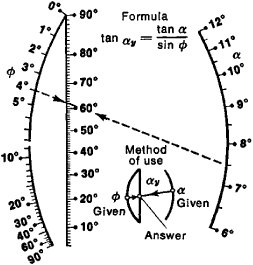Figure 1. Alignment chart for calculating αy

to the given values of a and ϕ must be found on the α and ϕ scales, and a straight line must be drawn through them. On the α y scale this line will pass through a point corresponding to the unknown value of αy. The broken line on the nomograph connects the points of the a and ϕ scales with the values α = 7.5° and ϕ = 4°; from the nomograph we obtain the answer αy = 62°.

Nomographs and their classification. Nomographs are classified according to the method of representation of the variables and the method of finding the correspondence between the representations of the variables.

REPRESENTATION OF VARIABLES. A variable is represented in a nomograph by a line or by a one-parameter family of curves. Certain values of the variable are identified by labelled points on the line or by labelled curves. The line or family of curves constitute a graduated scale for the variable, indicating its range of values. Values corresponding to unmarked points on the scale are established by linear interpolation, which is carried out on the nomograph by sight. Similarly, curves within a family that are not indicated in the nomograph are found by interpolation. In addition to one-dimensional scales, two-dimensional regions can be used to represent pairs of variables, each point in the region corresponding to a pair of values of the variables being represented. Two families of curves— u = const and v = const (in Figure 3, the lines h and curves ϕ)—are laid out in the region to make it possible to locate points on the basis of variable values, and vice versa. Linear interpolation can also be used here if necessary.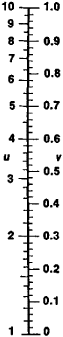Figure 2. Double scale for calculating the logarithm (u) of a number (v)

CLASSIFICATION OF NOMOGRAPHS. The following types of nomographs are most widely used: alignment charts, intersection charts, and transparency nomographs. Double scales are used for an equation in two variables.

The double scale is the simplest type of nomograph. For the equation F(u, v) = 0 it consists of combined scales for the variables u and v. The scales are constructed in such a way that the points corresponding to values satisfying the equation coincide.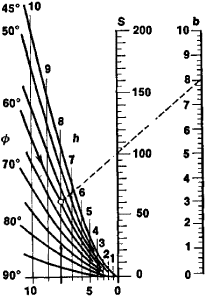Figure 3. Alignment chart with a binary field for calculating the area (S) of an isoscles trapezoid

Figure 2 illustrates a double scale for calculating logarithms: u = log v.

The alignment chart of the equation F(u, v, w) = 0 consists of three scales representing the ranges of the variables u, v, and w that represent the range of change of variables. The scales are constructed so that three values that satisfy the equation lie on a straight line (Figure 1 is an example of an alignment chart).

The alignment chart with a two-dimensional region for the equation F(u, v, w, t) = 0 in four variables consists of scales for the variables u and v and a region for the variables w and t. The nomograph scales and region are constructed so that the two points with the values u and v on the scales and the point in the region with the value-pair (w, t) lie on a straight line if the values of the variables u, v, w, and t satisfy the equation.

A nomograph with two scales and a region is illustrated in Figure 3. It is used to calculate the area S of an isosceles trapezoid with respect to the length b of the short base, altitude h, and angle ϕ between the long base and a side:

S = bh + h2 cot ϕ

The nomograph consists of scale S, scale b, and the region (ϕ, h). To find S, a point in the region must be found from the given h and ϕ, a point on the scale must be found from the given b, and a straight line must be drawn through these points. The value of the point of intersection of the line with scale S gives the answer. An example in which h = 8, ϕ = 60°, and b = 8 is illustrated in Figure 3. The answer is S = 100.

An alignment chart may contain two or three two-dimensional regions; that is, the solution of an equation in either five or six variables can be given by a single application of a ruler.

The intersection chart for the equation F(u, v, w) = 0 in three variables u, v, and w consists of three families of labelled curves that represent the given ranges of these variables. The curves of these families are constructed such that every three curves whose values satisfy the equation intersect at a single point. Figure 4 gives an example of an intersection chart for determining the necessary reactive power factor k per kilowatt of load on an electrical device to increase cos ϕ from cos ϕ1 to cos ϕ2, where k = tan ϕ1 — tan ϕ2. The nomograph consists of a family of straight lines labelled with the values of the initial cos ϕ1, a family of straight lines labelled with the values of k, and a family of curves labelled with the values of the unknown cos ϕ2. To calculate the quantity k from given cos ϕ1 and cos ϕ2 we must find the corresponding curves and their point of intersection on the nomograph. The value associated with the curve in the k family that passes through this point gives the answer; for example, for cos = 0.8 and cos ϕ2 = 0.95 (“lag”) we find k = 0.4.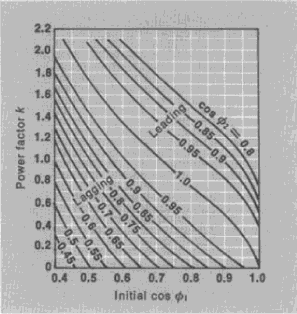Figure 4. Intersection chart for calculating the power required per kW of load of an electrical device for transition from cos ϕ1 to cos ϕ2

A problem that can be posed in the construction of an intersection chart is to find a transformation such that all three families of curves on the nomograph are reduced to families of straight lines, which simplifies the drawing of the nomograph.

Such a transformation is called an anamorphosis and is equivalent to the problem of constructing an alignment chart for a given equation, since an intersection chart consisting of straight lines can be converted into an alignment chart with three scales by a dualization transformation (in which three concurrent lines are transformed into three collinear points). So-called functional grids are used to construct intersection charts of straight lines. A functional grid is a system of coordinates (u, v) (often rendered typographically) in which the (x, y)-coordinates of a point are related to the values (u, v) by the transformations

x = ϕ1 (u), y = ϕ2(v)

Logarithmic and semilogarithmic paper are the simplest functional grids. Similarly, grids can be constructed in which parts of a sine curve or the normal probability distribution are represented by straight lines. Functional grids are also used to construct intersection charts, when the curves of the third family are simpler or more graphic on a grid than in a Cartesian coordinate system.

A transparency nomograph, in the simplest case, consists of two planes—the primary plane and a transparent plane with representations of variables in the form of scales, regions, or families of labelled curves; the primary plane and transparency may also contain unmarked (“mute”) lines and points. The nomograph is constructed in such a way that elements marked with values that satisfy the equation, as well as “mute” elements of the nomograph, are in contact in a definite sequence when the transparency is placed over the primary plane. When these two elements are in contact, one is said to belong to the other (for example, the point lies on the curve or the line touches the curve). When necessary, the transparency is made of a transparent material to ensure the required contacts.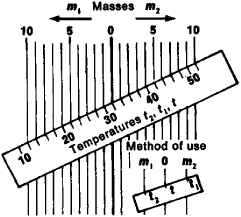Figure 5. Transparency nomograph for determining the temperature of a mixture of two liquids with identical heat capacities

Figure 5 illustrates a transparency nomograph for calculating the temperature t of a mixture of two liquids with identical heat capacities by the formula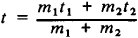where m1 is a mass with temperature t1 and m2 is a mass with temperature t2. The nomograph consists of a family of parallel lines on the primary plane of the nomograph and a scale on a transparency marked like a ruler. The lines to the left of the central line marked 0 (the darker line in Figure 5) represent m1, and the lines to the right of the central line represent m2. The scale of the transparency is at the same time a scale for the variables t1, t2, and t. In order to use the nomograph for calculation, the transparency is placed over the primary plane in such a way that the points corresponding to m1 and m2 are on lines corresponding to the t2 and t1; that is, the point t2 and the line m1 and the point t1 and the line m2 are in contact. The answer will be the value on the t scale at the point of intersection with the line marked 0 on the primary plane. This line, in this case, serves as the unmarked element that is in contact with the point on the answer scale. An example in which m1 = 8 kg, t1 = 52°, m2 = 10 kg, and t2 = 16° is solved in Figure 5. The answer is t = 32°.

A slide rule is an example of a transparency nomograph whose transparency has only reciprocating motion.

COMPOSITE NOMOGRAPHS. Composite nomographs, which are systems of individual nomographs connected by common scales or families of curves, are used for equations in many variables. Alignment and intersection charts are usually the elements of composite nomographs.

Errors in nomographic calculations. Nomographic calculations are accompanied by errors that result from the impossibility of achieving precisely, in the process of calculation, the necessary correspondence between elements of the nomograph.

The accuracy of nomographic calculations depends significantly on how well the necessary operations are carried out. In making calculations based on alignment charts, a transparent ruler with a longitudinal guide line should be used.

Possibility of representing equations as nomographs. Nomographs are divided into exact and approximate.

A nomograph with a given functional relation is said to be exact if the correspondence that it provides between representations of variables (on the assumption that they are accurately plotted) establishes a dependence between variables that is coincident with the given relation.

The conditions for exact nomography impose certain restrictions on the type of equations suitable for nomographs.

The conditions that an equation must satisfy in order that a nomograph be constructed are called the conditions of nomo-graphability. In constructing nomographs, the equation to be nomographed is transformed into canonical form, for which the equations of the scales, fields, and families of curves of the corresponding nomograph are known in general form.

In constructing composite nomographs, the representation of a given equation in many variables in the form of a system of equations with a smaller number of variables—the so-called separation for division—is also necessary; this is accomplished by introducing auxiliary parameters.

A nomograph of a given functional relation is said to be approximate if the correspondence that it achieves between its elements (on the assumption of accurate plotting) establishes a relation between the variables that approximately represents the given relation. A number of methods have been developed for constructing approximate nomographs, most of which are alignment charts.

The approximate nomograph of the integral law of Student’s t-distribution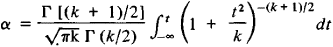is presented in Figure 6. The error in the determination of t owing to approximate nomography in the given range of change of variables α, k, and t does not exceed ±0.001.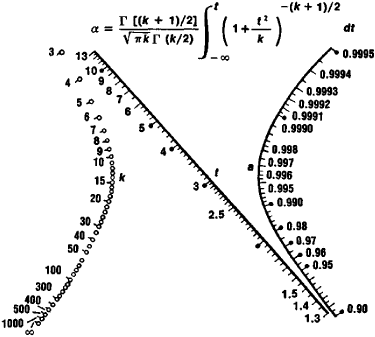Figure 6. Approximate alignment chart of Student’s t-distribution

Approximate nomographs are used when exact nomographs are impossible or when exact nomographs have an unsuitable form and produce a larger error in the answer.

History. Geometric representations of relations between variables in order to eliminate calculations have long been known. They include quite complex constructs that contain families of curves and scales as representations of variables; these are encountered, for example, in sundials and astrolabes. The theory of nomograph construction first emerged in the 19th century with the creation of the theory of construction of rectilinear grid nomographs by the French mathematician L.-L.-C. Lalanne (1843). The principles of the general theory of nomograph construction were given by M. d’Ocagne (1884–91), who also introduced the term “nomography.” In the period 1906–08, N. M. Gersevanov was the first person in Russia to work on problems of nomography. A major contribution to the development of the theory of nomography and the organization of nomography for engineering calculations was made by N. A. Glagolev, who led the Soviet nomographic school.

### REFERENCES

Pentkovskii, M. V. Schitaiushchie chertezhi (Nomogrammy), 2nd ed. Moscow, 1959.
Pentkovskii, M. V. Nomografiia. Moscow-Leningrad, 1949.
Gersevanov, N. M. Osnovy nomografii, 2nd ed. Moscow-Leningrad, 1932.
Glagolev, N. A. Teoreticheskie osnovy nomografii, 2nd ed. Moscow-Leningrad, 1936.
Glagolev, N. A. Kurs nomografii, 2nd ed. Moscow, 1961.
Nevskii, B. A. Spravochnaia kniga po nomografii. Moscow-Leningrad, 1951.
Nomograficheskii sbornik. Moscow, 1951.
D’Ocagne, M. Traité de nomographie, 2nd ed. Paris, 1921.
Soureau, R. Nomographie ou traité des abaques, vols. 1–2. Paris, 1921.

M. V. PENTKOVSKII

Site: Follow: Share:
Open / Close